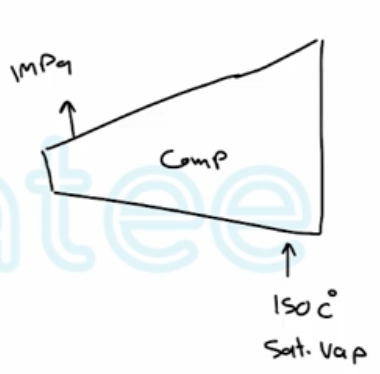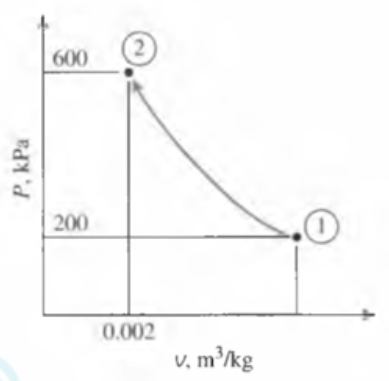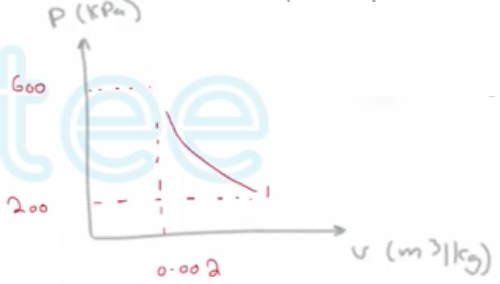Need Help?

Subscribe to Thermodynamics

###### \${selected_topic_name}
• Notes

$\begin{array}{l}{\text { Saturated water vapor at } 150^{\circ} \mathrm{C} \text { is compressed in a reversible steady-flow device to } 1000 \mathrm{kPa}} \\ {\text { while its specific volume remains constant. Determine the work required, in } \mathrm{k} / \mathrm{kg} \text { . }}\end{array}$$\left.\begin{array}{l}{T_{1}=150 c^{\circ}} \\ {X_{1}=1}\end{array}\right] \rightarrow A-4$

$∴ P_{1}=476.16 \mathrm{kPa}$

$v_{1}=0.39248 \mathrm{m}^{3} / \mathrm{Kg}$

$W_{i n}=\int_{1}^{2} v d P=V_{1}\left(P_{2}-P_{1}\right)$

$=0.39248(1000-476.16)$

$=205 .6 \mathrm{kJ} / \mathrm{kg}$

$\begin{array}{l}{\text { Calculate the work produced, in } \mathrm{kJ} / \mathrm{kg} \text { , for the reversible isothermal, steady-flow process } 1-3} \\ {\text { shown in Fig. } \mathrm{P} 7-110 \text { when the working fluid is an ideal gas. }}\end{array}$$W_{12}=\int_{1}^{2} v d P$                                            $v= \frac{R T}{P}$

$=\int_{1}^{2} R \frac{ T}{p} d p$

$=-R T \int_{1}^{2} \frac{d P}{P}$

$=-R T \ln \frac{P_{2}}{P_{1}}=-P_{2} V_{2} \ln \frac{P_{2}}{P_{1}}$

$=-(600)(0.002) \ln \frac{600}{200}$

$=-1.32 \ \mathrm{kJ} / \mathrm{kg}$